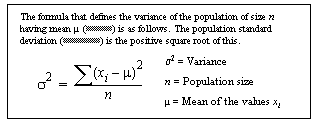# stddev_pop

Computes the standard deviation of a population consisting of a numeric expression, as a double. stdevp is an alias for stddev_pop, and uses the same syntax.

## Syntax

`stddev_pop ( [ all | distinct ] expression )`

## Parameters

• all – applies stddev_pop to all values. all is the default.
• distinct – eliminates duplicate values before stddev_pop is applied.
• expression – is the expression—commonly a column name—in which its population-based standard deviation is calculated over a set of rows.

## Examples

• Example 1 – The following statement lists the average and standard deviation of the advances for each type of book in the pubs2 database.
```select type, avg(advance) as "avg", stddev_pop(advance)
as "stddev" from titles group by type order by type```

## Usage

Computes the population standard deviation of the provided value expression evaluated for each row of the group (if distinct was specified, then each row that remains after duplicates have been eliminated), defined as the square root of the population variance.

Formula for Population-Related Statistical Aggregate Functions